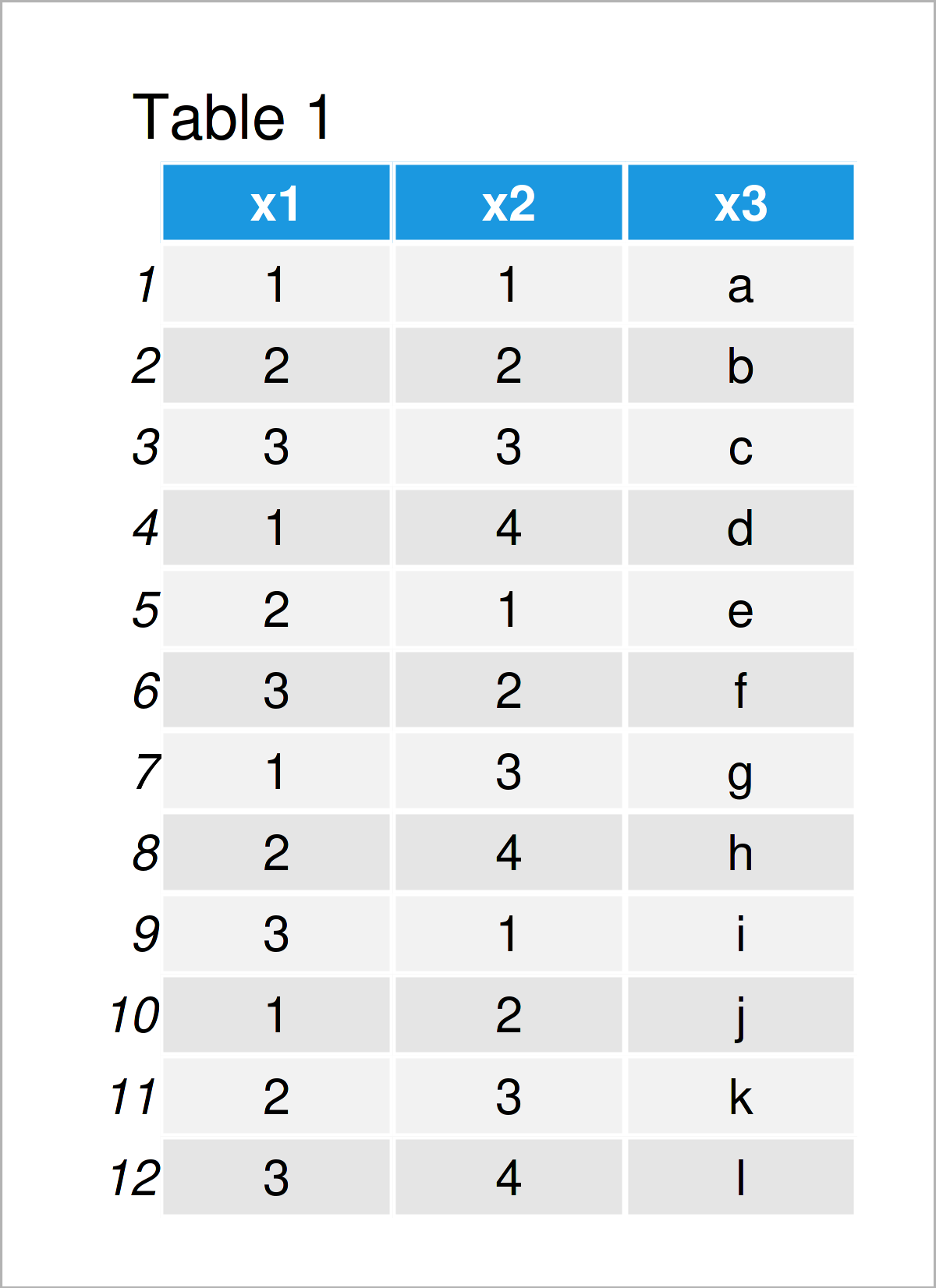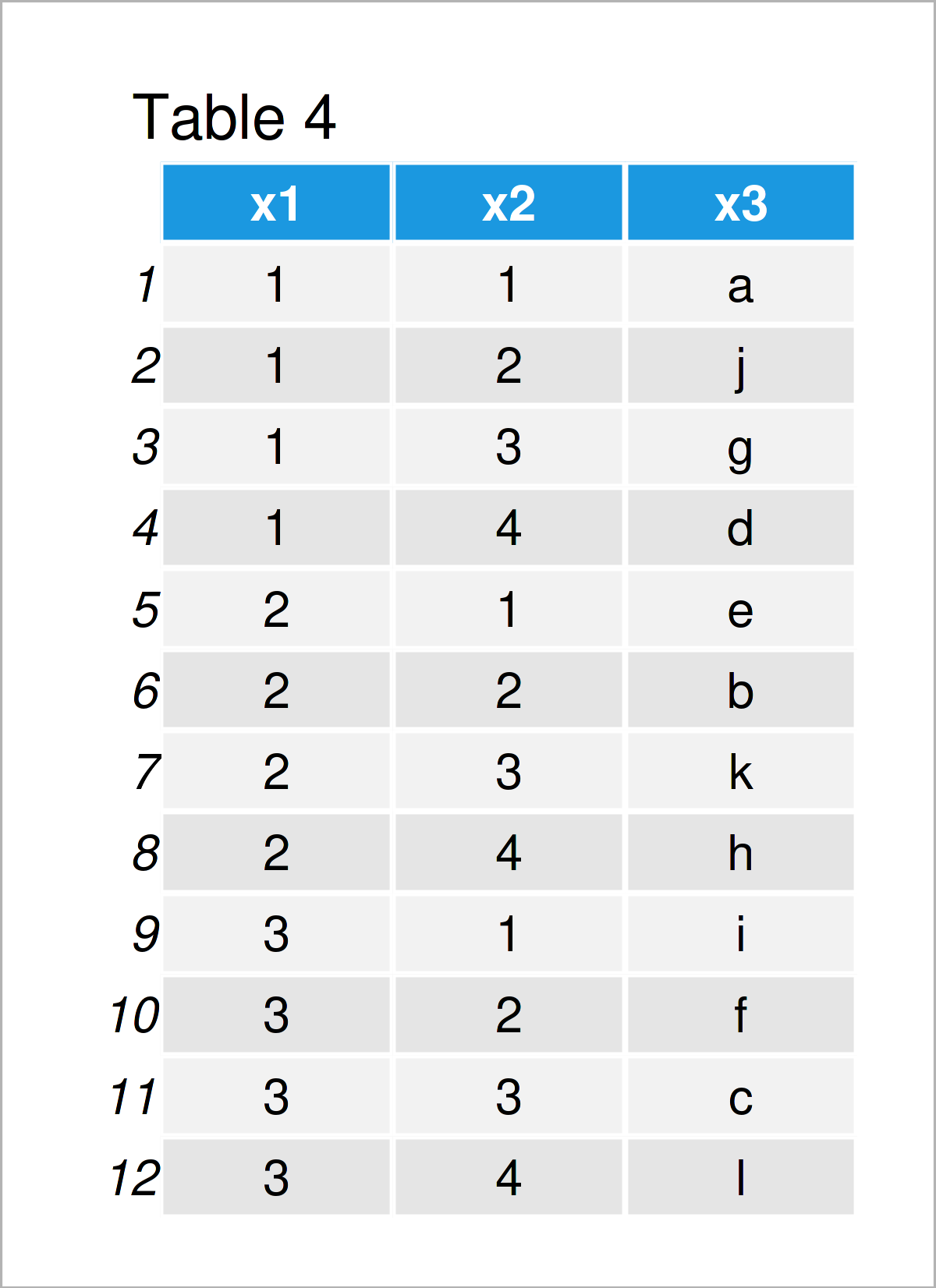# Sort Column Based On Other Variable in R (3 Examples)

In this article, I’ll show how to order a data frame variable based on another column in the R programming language.

The article consists of three examples for the ordering of the column of a data frame based on another column. To be more specific, the content of the post is structured as follows:

You’re here for the answer, so let’s get straight to the exemplifying R syntax…

## Creating Example Data

As the first step, we’ll need to create some data that we can use in the following examples:

```data <- data.frame(x1 = 1:3,                     # Create example data frame
x2 = 1:4,
x3 = letters[1:12])
data                                             # Print example data frame```As you can see based on Table 1, our example data is a data frame constituted of twelve data points and three columns. The variables x1 and x2 have the integer class and the column x3 has the character class.

## Example 1: Sort Data Frame Based On Single Column Using order() Function

The following syntax shows how to order the rows of a data frame based on the values in a single column.

For this task, we can apply the order function as shown below:

```data_sort1 <- data[order(data\$x1), ]             # Order data based on one column
data_sort1```Table 2 shows the output of the previous R programming code – We have sorted our data frame based on the column x1.

## Example 2: Sort Data Frame Based On Multiple Columns Using order() Function

In Example 2, I’ll illustrate how to sort the columns in a data frame based on multiple sorting variables.

Once again, we can apply the order function for this task:

```data_sort2 <- data[order(data\$x1, data\$x2), ]    # Order data based on multiple columns
data_sort2```Table 3 shows the output of the previous R code – We have ordered our data matrix based on the columns x1 and x2.

## Example 3: Sort Data Frame Based On Multiple Columns Using arrange() Function of dplyr Package

Example 3 explains how to use the functions of the dplyr package to sort a data frame column by other columns.

First, we need to install and load the dplyr package:

```install.packages("dplyr")                        # Install dplyr package

In the next step, we can apply the arrange function to order our data frame:

```data_sort3 <- data %>%                           # Order data using dplyr package
arrange(x1, x2)
data_sort3```After executing the previous R programming syntax the data frame shown in Table 4 has been created.

The ordering is the same as in Example 2. However, this time we have used the dplyr package instead of Base R.

## Video, Further Resources & Summary

Have a look at the following video on my YouTube channel. In the video, I show the R programming syntax of this article.

In addition, you could have a look at the other tutorials on my website.

In this article, I have demonstrated how to sort a data frame variable based on another column in the R programming language. In case you have additional questions, kindly let me know in the comments section.

Subscribe to the Statistics Globe Newsletter